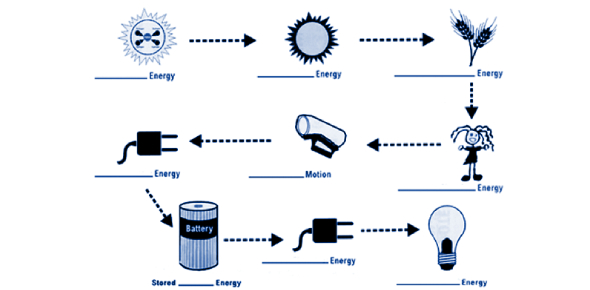# The Energy Transfer Section Of Unit 1

10 Questions | Total Attempts: 5136Settings• 1.
Thermal energy that is transferred from matter at a higher temperature to matter at a lower temperature is called
• A.

Kinetic energy

• B.

Heat

• C.

Temperature

• 2.
Heat that is transerred from one particle of matter to another without the movement of matter is
• A.

Conduction

• B.

Convection

• C.

• 3.
Heat that is trasnferred by the movement of currents within a fluid is
• A.

Conduction

• B.

Convection

• C.

• 4.
Heat that is transferred by electromagnetic radiation is called
• A.

Conduction

• B.

Convection

• C.

• 5.
A turtle basking in the sun is an example of
• A.

Conduction

• B.

Convection

• C.

• 6.
Opening the windows in a hot room is an example of
• A.

Conduction

• B.

Convection

• C.

• 7.
Putting a ice on a sprained ankle is an example of
• A.

Conduction

• B.

Convection

• C.

• 8.
A lava lamp does an excellent job demonstrating convection currents.  What happens to the lava when it heats up?
• A.

Lava becomes less dense and rises

• B.

Lava becomes more dense and rises

• C.

Lava becomes less dense and sinks

• D.

Lava become more dense and sinks.

• 9.
How is heat transferred?
• A.

Heat is transferred from a warmer object to a cooler object.

• B.

Heat is transfferred from a cooler object to a warmer object.

• C.

Heat moves simultaneously through both objects.

• 10.
How does a glass of lemonade become cold when you put ice in it?
• A.

Heat energy from the lemonade is transferred to the ice cube.

• B.

Heat energy from the ice cube is transferred to the lemonade.

• C.

Heat energy from the ice cube makes the lemonade cold.

Related TopicsBack to top